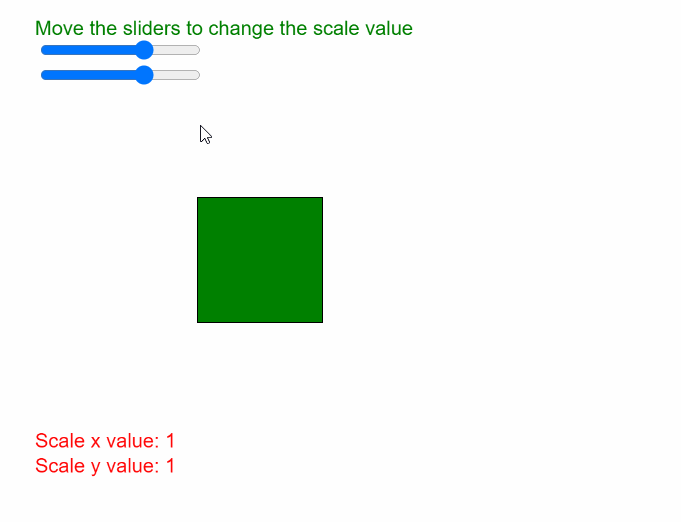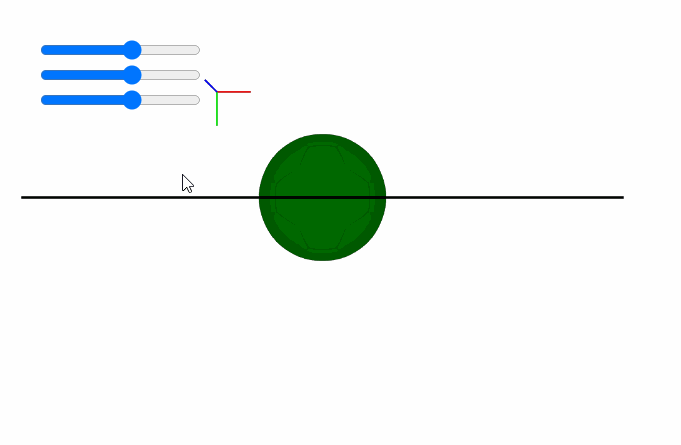Open in App
Not now

# p5.js | scale() Function

• Last Updated : 28 May, 2020

The scale() function in p5.js is used to increase or decrease the size of a shape or model by expanding or contracting its vertices. The scale values are specified as decimal percentages, that is, a scale value of “2.0” would increase the dimensions of the shape by 200%. Similarly, a negative of “-2.0” would decrease the dimensions of the shape by 200%

The objects always scale from their relative origin to the coordinate system. The z parameter of this function is only available in the WebGL mode for scaling across the z-axis.

As `scale()` is a transformation, every transformation that happens after one call multiplies the effect. If `scale()` is called within the `draw()` loop, then the transformation is reset when the loop begins again.

Syntax:

`scale( s, [y], [z] )`

OR

`scale( scales )`

Parameters: This function accepts four parameters as mentioned above and described below.

• s: It is a p5.Vector, Number or array of Numbers which define the percent to scale the object. If multiple arguments are given, it denotes the percent to scale the object in the x direction.
• y: It is a Number which denotes the percent to scale the object in the y direction. It is an optional parameter.
• z: It is a Number which denotes the percent to scale the object in the z direction. It is an optional parameter.
• scales: It is a p5.Vector or array of Numbers which specify per-axis percents to scale the object.

The program below illustrate the scale() function in p5.js:

Example 1:

 `function` `setup() { ` `  ``createCanvas(500, 400); ` `  ``textSize(16); ` ` `  `  ``scaleXslider = createSlider(-3, 3, 1, 0.1); ` `  ``scaleXslider.position(30, 30); ` ` `  `  ``scaleYslider = createSlider(-3, 3, 1, 0.1); ` `  ``scaleYslider.position(30, 50); ` `} ` ` `  `function` `draw() { ` `  ``clear(); ` `  ``text(``"Move the sliders to change the scale value"``, 20, 20); ` `  ``fill(``"red"``); ` `  ``rect(150, 150, 100, 100); ` ` `  `  ``// Get the scale parameters ` `  ``let scaleXValue = scaleXslider.value(); ` `  ``let scaleYValue = scaleYslider.value(); ` ` `  `  ``text(``"Scale x value: "` `+ scaleXValue, 20, 350); ` `  ``text(``"Scale y value: "` `+ scaleYValue, 20, 370); ` ` `  `  ``// Set the scale according to properties ` `  ``scale(scaleXValue, scaleYValue); ` ` `  `  ``fill(``"green"``); ` `  ``rect(150, 150, 100, 100); ` `} `

Output:Example 2:

 `function` `preload() { ` `  ``ballObj = loadModel(``"models/ball.obj"``, ``true``); ` `} ` ` `  `function` `setup() { ` `  ``createCanvas(500, 300, WEBGL); ` ` `  `  ``scaleXslider = createSlider(-3, 3, 0.5, 0.1); ` `  ``scaleXslider.position(30, 30); ` ` `  `  ``scaleYslider = createSlider(-3, 3, 0.5, 0.1); ` `  ``scaleYslider.position(30, 50); ` ` `  `  ``scaleZslider = createSlider(-3, 3, 0.5, 0.1); ` `  ``scaleZslider.position(30, 70); ` ` `  `  ``debugMode(); ` `} ` ` `  `function` `draw() { ` `  ``clear(); ` `  ``noStroke(); ` `  ``lights(); ` `  ``orbitControl(); ` ` `  `  ``// Get the scale parameters ` `  ``let scaleXValue = scaleXslider.value(); ` `  ``let scaleYValue = scaleYslider.value(); ` `  ``let scaleZValue = scaleZslider.value(); ` ` `  `  ``// Set the scale according to properties ` `  ``scale(scaleXValue, scaleYValue, scaleZValue); ` ` `  `  ``fill(``"green"``) ` `  ``model(ballObj); ` `} `

Output:Reference: https://p5js.org/reference/#/p5/scale

My Personal Notes arrow_drop_up
Related Articles